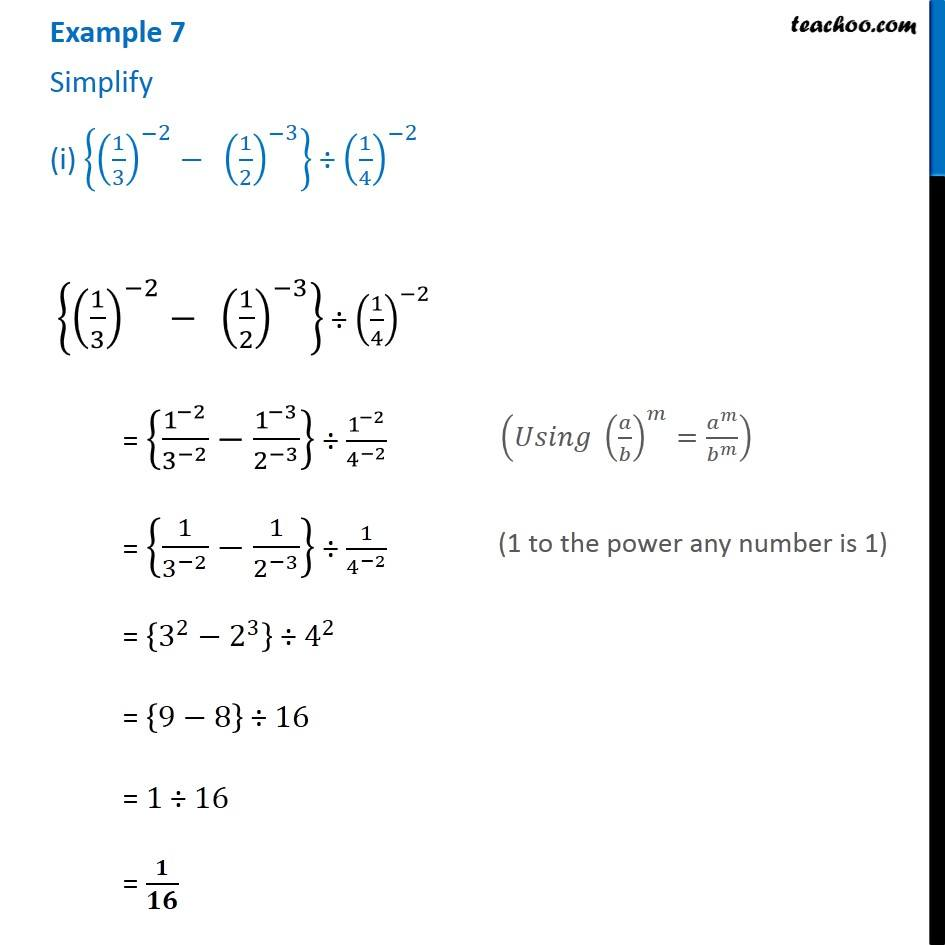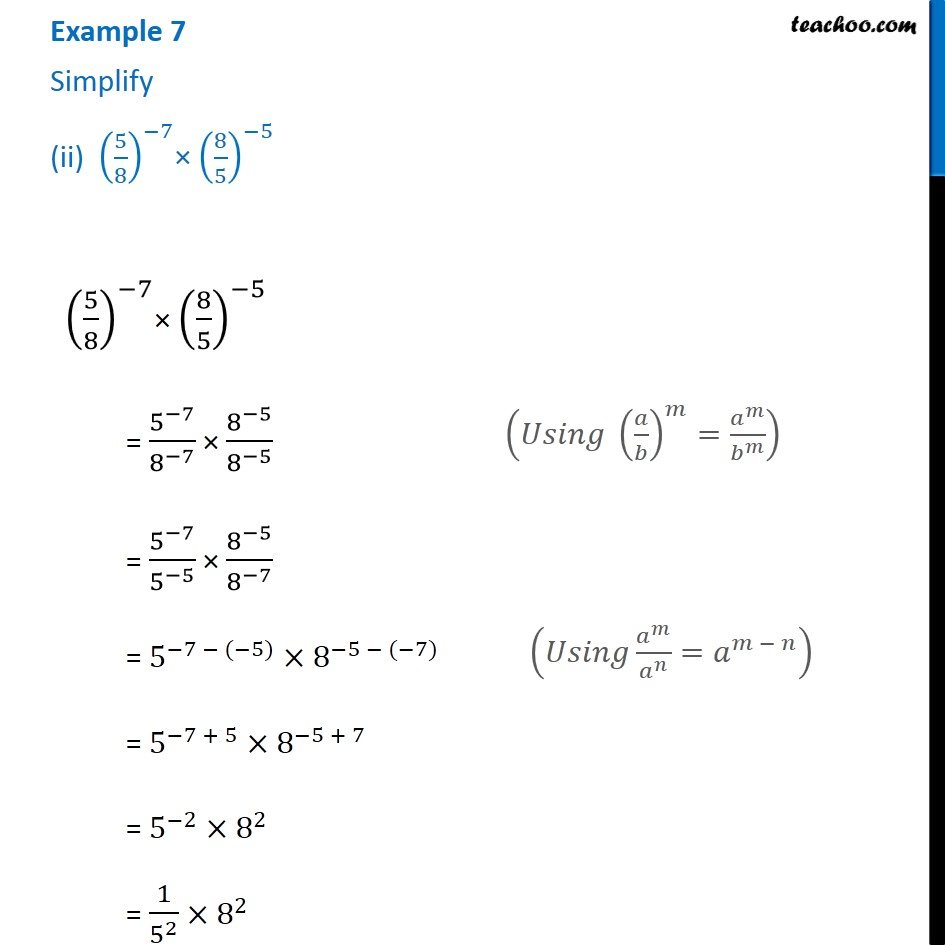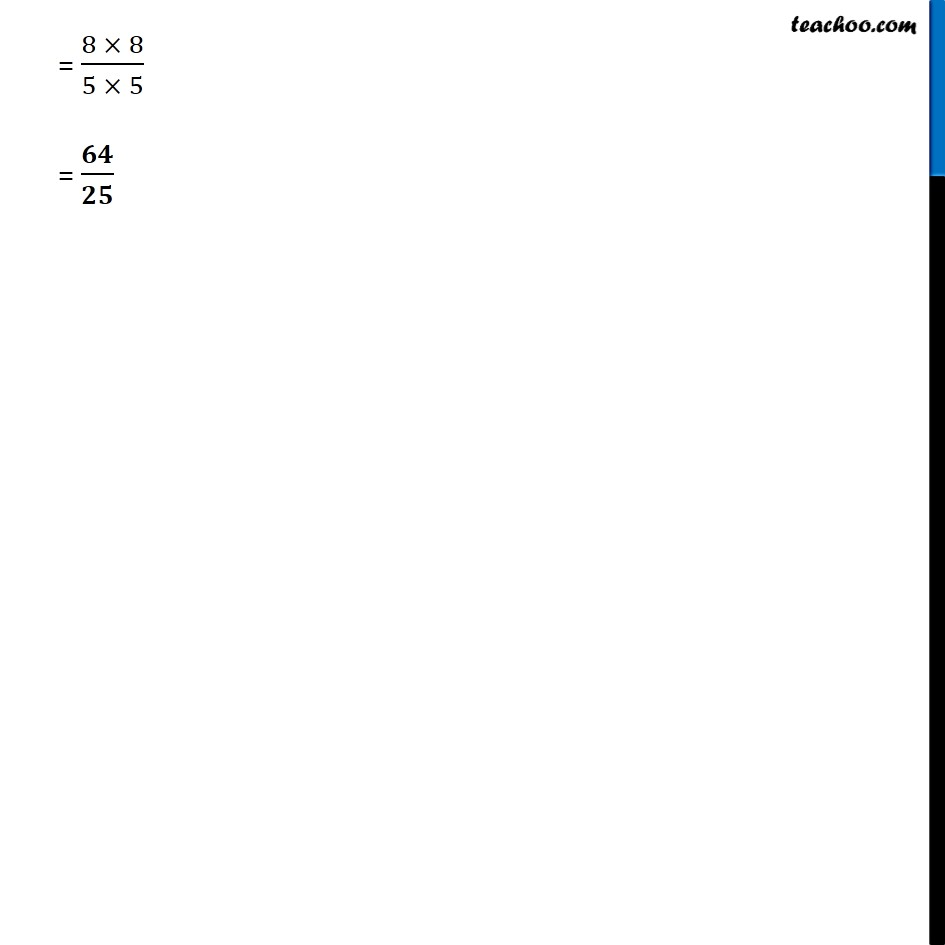1. Chapter 12 Class 8 Exponents and Powers
2. Serial order wise
3. Examples

Transcript

Example 7 Simplify (i) {(1/3)^(−2)−" " (1/2)^(−3) } ÷ (1/4)^(−2) {(1/3)^(−2)−" " (1/2)^(−3) } ÷ (1/4)^(−2) = {1^(−2)/3^(−2) −1^(−3)/2^(−3) } ÷ 1^(−2)/4^(−2) = {1/3^(−2) −1/2^(−3) } ÷ 1/4^(−2) = {3^2−2^3 } ÷ 4^2 = {9−8} ÷ 16 = 1 ÷ 16 = 𝟏/𝟏𝟔 (𝑈𝑠𝑖𝑛𝑔 (𝑎/𝑏)^𝑚=𝑎^𝑚/𝑏^𝑚 ) (1 to the power any number is 1) Example 7 Simplify (ii) (5/8)^(−7)× (8/5)^(−5) (5/8)^(−7)× (8/5)^(−5) = 5^(−7)/8^(−7) × 8^(−5)/8^(−5) = 5^(−7)/5^(−5) × 8^(−5)/8^(−7) = 5^(−7 − (−5))×8^(−5 − (−7)) = 5^(−7 + 5)×8^(−5 + 7) = 5^(−2)×8^2 = 1/5^2 ×8^2 (𝑈𝑠𝑖𝑛𝑔 (𝑎/𝑏)^𝑚=𝑎^𝑚/𝑏^𝑚 ) (𝑈𝑠𝑖𝑛𝑔 𝑎^𝑚/𝑎^𝑛 =𝑎^(𝑚 − 𝑛) ) = (8 × 8)/(5 × 5) = 𝟔𝟒/𝟐𝟓

Examples

About the AuthorDavneet Singh
Davneet Singh is a graduate from Indian Institute of Technology, Kanpur. He has been teaching from the past 10 years. He provides courses for Maths and Science at Teachoo.